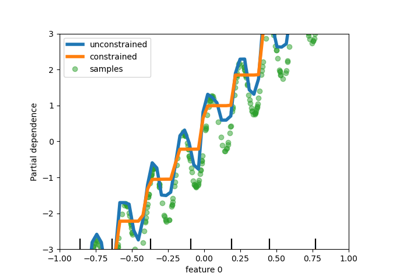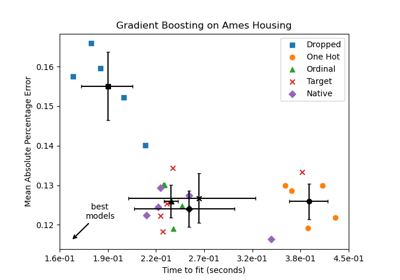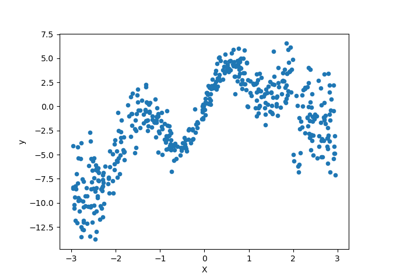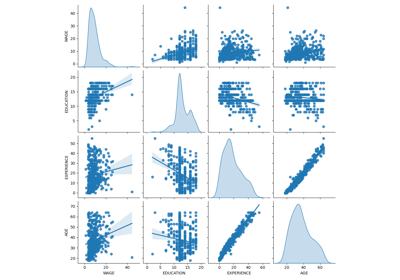# sklearn.compose.make_column_transformer¶

sklearn.compose.make_column_transformer(*transformers, remainder='drop', sparse_threshold=0.3, n_jobs=None, verbose=False)[source]

Construct a ColumnTransformer from the given transformers.

This is a shorthand for the ColumnTransformer constructor; it does not require, and does not permit, naming the transformers. Instead, they will be given names automatically based on their types. It also does not allow weighting with transformer_weights.

Read more in the User Guide.

Parameters
*transformerstuples

Tuples of the form (transformer, columns) specifying the transformer objects to be applied to subsets of the data.

transformer{‘drop’, ‘passthrough’} or estimator

Estimator must support fit and transform. Special-cased strings ‘drop’ and ‘passthrough’ are accepted as well, to indicate to drop the columns or to pass them through untransformed, respectively.

columnsstr, array-like of str, int, array-like of int, slice, array-like of bool or callable

Indexes the data on its second axis. Integers are interpreted as positional columns, while strings can reference DataFrame columns by name. A scalar string or int should be used where transformer expects X to be a 1d array-like (vector), otherwise a 2d array will be passed to the transformer. A callable is passed the input data X and can return any of the above. To select multiple columns by name or dtype, you can use make_column_selector.

remainder{‘drop’, ‘passthrough’} or estimator, default=’drop’

By default, only the specified columns in transformers are transformed and combined in the output, and the non-specified columns are dropped. (default of 'drop'). By specifying remainder='passthrough', all remaining columns that were not specified in transformers will be automatically passed through. This subset of columns is concatenated with the output of the transformers. By setting remainder to be an estimator, the remaining non-specified columns will use the remainder estimator. The estimator must support fit and transform.

sparse_thresholdfloat, default=0.3

If the transformed output consists of a mix of sparse and dense data, it will be stacked as a sparse matrix if the density is lower than this value. Use sparse_threshold=0 to always return dense. When the transformed output consists of all sparse or all dense data, the stacked result will be sparse or dense, respectively, and this keyword will be ignored.

n_jobsint, default=None

Number of jobs to run in parallel. None means 1 unless in a joblib.parallel_backend context. -1 means using all processors. See Glossary for more details.

verbosebool, default=False

If True, the time elapsed while fitting each transformer will be printed as it is completed.

Returns
ctColumnTransformer

ColumnTransformer

Class that allows combining the outputs of multiple transformer objects used on column subsets of the data into a single feature space.

Examples

>>> from sklearn.preprocessing import StandardScaler, OneHotEncoder
>>> from sklearn.compose import make_column_transformer
>>> make_column_transformer(
...     (StandardScaler(), ['numerical_column']),
...     (OneHotEncoder(), ['categorical_column']))
ColumnTransformer(transformers=[('standardscaler', StandardScaler(...),
['numerical_column']),
('onehotencoder', OneHotEncoder(...),
['categorical_column'])])


## Examples using sklearn.compose.make_column_transformer¶